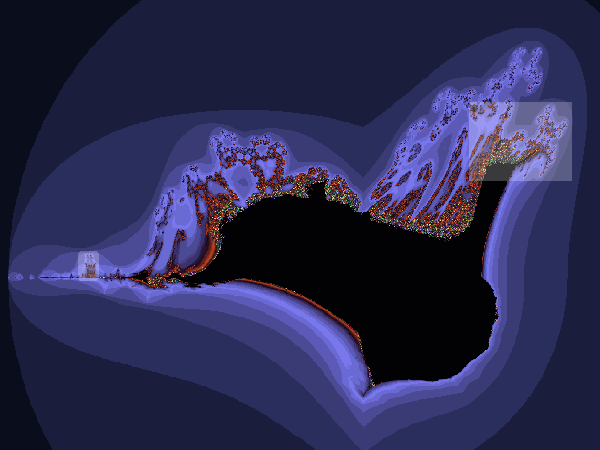Theory.org » fracdyn » buffalo » Buffalo Fractal

 A derivative of the Burning Ship, the Buffalo fractal was composed as follows: Let,  z0 = 0  zn+1 = zn2 - zn + c Take,  z = x + i*y, Then,  z2 - z + c = (x + i*y)2 - (x + i*y) + c Lastly, take the absolute values of x and y:  (|x| + i*|y|)2 - (|x| + i*|y|) + c